/ bezier Public

Helper for Bézier Curves, Triangles, and Higher Order Objects

# dhermes/bezier

Switch branches/tags
Nothing to show

A tag already exists with the provided branch name. Many Git commands accept both tag and branch names, so creating this branch may cause unexpected behavior. Are you sure you want to create this branch?

## Files

Failed to load latest commit information.

# `bezier`

Helper for Bézier Curves, Triangles, and Higher Order Objects

This library provides:

Dive in and take a look!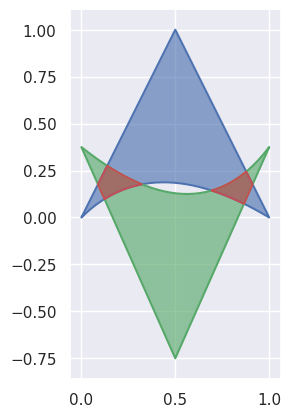## Why Bézier?

A Bézier curve (and triangle, etc.) is a parametric curve that uses the Bernstein basis: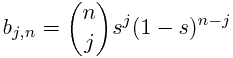to define a curve as a linear combination: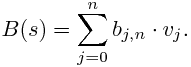This comes from the fact that the weights sum to one: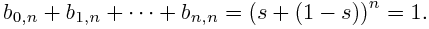This can be generalized to higher order by considering three, four, etc. non-negative weights that sum to one (in the above we have the two non-negative weights `s` and `1 - s`).

Due to their simple form, Bézier curves:

• can easily model geometric objects as parametric curves, triangles, etc.
• can be computed in an efficient and numerically stable way via de Casteljau's algorithm
• can utilize convex optimization techniques for many algorithms (such as curve-curve intersection), since curves (and triangles, etc.) are convex combinations of the basis

Many applications -- as well as the history of their development -- are described in "The Bernstein polynomial basis: A centennial retrospective", for example;

• aids physical analysis using finite element methods (FEM) on isogeometric models by using geometric shape functions called NURBS to represent data
• used in robust control of dynamic systems; utilizes convexity to create a hull of curves

## Installing

The `bezier` Python package can be installed with pip:

```\$ python     -m pip install --upgrade bezier
\$ python3.11 -m pip install --upgrade bezier
\$ # To install optional dependencies, e.g. SymPy
\$ python     -m pip install --upgrade bezier[full]```

To install a pure Python version (i.e. with no binary extension):

```\$ BEZIER_NO_EXTENSION=true \
>   python   -m pip install --upgrade bezier --no-binary=bezier```

`bezier` is open-source, so you can alternatively grab the source code from GitHub and install from source.

## Getting Started

For example, to create a curve:

```>>> import bezier
>>> import numpy as np
>>> nodes1 = np.asfortranarray([
...     [0.0, 0.5, 1.0],
...     [0.0, 1.0, 0.0],
... ])
>>> curve1 = bezier.Curve(nodes1, degree=2)```

The intersection (points) between two curves can also be determined:

```>>> nodes2 = np.asfortranarray([
...     [0.0, 0.25,  0.5, 0.75, 1.0],
...     [0.0, 2.0 , -2.0, 2.0 , 0.0],
... ])
>>> curve2 = bezier.Curve.from_nodes(nodes2)
>>> intersections = curve1.intersect(curve2)
>>> intersections
array([[0.31101776, 0.68898224, 0. , 1. ],
[0.31101776, 0.68898224, 0. , 1. ]])
>>> s_vals = np.asfortranarray(intersections[0, :])
>>> points = curve1.evaluate_multi(s_vals)
>>> points
array([[0.31101776, 0.68898224, 0. , 1. ],
[0.42857143, 0.42857143, 0. , 0. ]])```

and then we can plot these curves (along with their intersections):

```>>> import seaborn
>>> seaborn.set()
>>>
>>> ax = curve1.plot(num_pts=256)
>>> _ = curve2.plot(num_pts=256, ax=ax)
>>> lines = ax.plot(
...     points[0, :], points[1, :],
...     marker="o", linestyle="None", color="black")
>>> _ = ax.axis("scaled")
>>> _ = ax.set_xlim(-0.125, 1.125)
>>> _ = ax.set_ylim(-0.0625, 0.625)```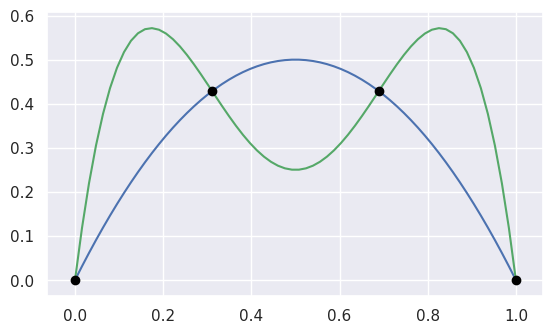For API-level documentation, check out the Bézier Python package documentation.

## Development

To work on adding a feature or to run the functional tests, see the DEVELOPMENT doc for more information on how to get started.

## Citation

For publications that use `bezier`, there is a JOSS paper that can be cited. The following BibTeX entry can be used:

```@article{Hermes2017,
doi = {10.21105/joss.00267},
url = {https://doi.org/10.21105%2Fjoss.00267},
year = {2017},
month = {Aug},
publisher = {The Open Journal},
volume = {2},
number = {16},
pages = {267},
author = {Danny Hermes},
title = {Helper for B{\'{e}}zier Curves, Triangles, and Higher Order Objects},
journal = {The Journal of Open Source Software}
}```

A particular version of this library can be cited via a Zenodo DOI; see a full list by version.

`bezier` is made available under the Apache 2.0 License. For more details, see the LICENSE.

Helper for Bézier Curves, Triangles, and Higher Order Objects

2021.2.12 Latest
Feb 14, 2021

## Packages 0

No packages published